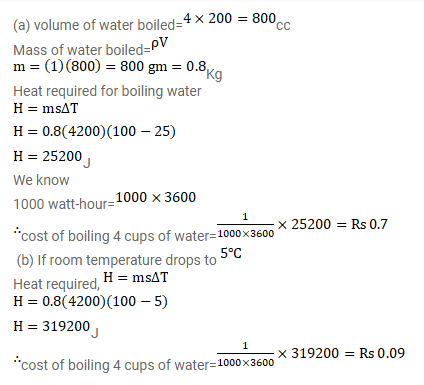# An electric kettle used to prepare tea,

Question:

An electric kettle used to prepare tea, takes 2 minutes to boil 4 cups of water ( 1 cup contains 200 cc of water) is the room temperature is $25^{\circ} \mathrm{C}$. (a) If the cost of power consumption is Rs $1.00$ per unit ( 1 unit=1000 watt-hour), calculate the cost of boiling 4 cups of water. (b) What will be the corresponding cost if the room temperature drops to $5^{\circ} \mathrm{C}_{\text {? }}$ ?

Solution: